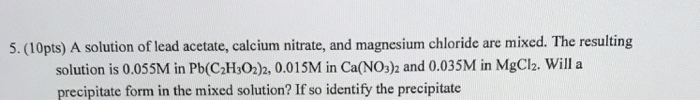# Question & Answer: A solution of lead acetate, calcium nitrate, and magnesium chloride are mixed. The resulting…..A solution of lead acetate, calcium nitrate, and magnesium chloride are mixed. The resulting solution is 0.055M in Pb(C_2H_3O_2)_2, 0.015M in Ca(NO_3)_2 and 0.035M in MgCl_2. Will a precipitate form in the mixed solution? If so identify the precipitate

Yes , precipitate will form in the mixed solution

The precipitate is PbCl2

Don't use plagiarized sources. Get Your Custom Essay on
Question & Answer: A solution of lead acetate, calcium nitrate, and magnesium chloride are mixed. The resulting…..
GET AN ESSAY WRITTEN FOR YOU FROM AS LOW AS \$13/PAGE

Explanation

The equillibrium involved in solubility of PbCl2 is

PbCl2(s) <——-> Pb2+(aq) + 2Cl-(aq)

solubility product, Ksp = [ Pb2+ ] [ Cl- ]^2 = 1.7×10^-4

solubility product quotient,

Qsp = [ Pb2+ ] × [ Cl- ]^2

[ Pb( C2H3O2)2] = 0.055M

Pb(C2H3O2)2 contain 1 Pb

So , [ Pb2+ ] = 0.055M

[ MgCl2 ] = 0.035M

MgCl2 contain 2Cl

so, [ Cl- ] = 0.070M

Therefore,

Qsp = (0.055) ×(0.070)^2

= 2.7×10^-4

So, Qsp > Ksp

Therefore, precipitation of PbCl2 will form